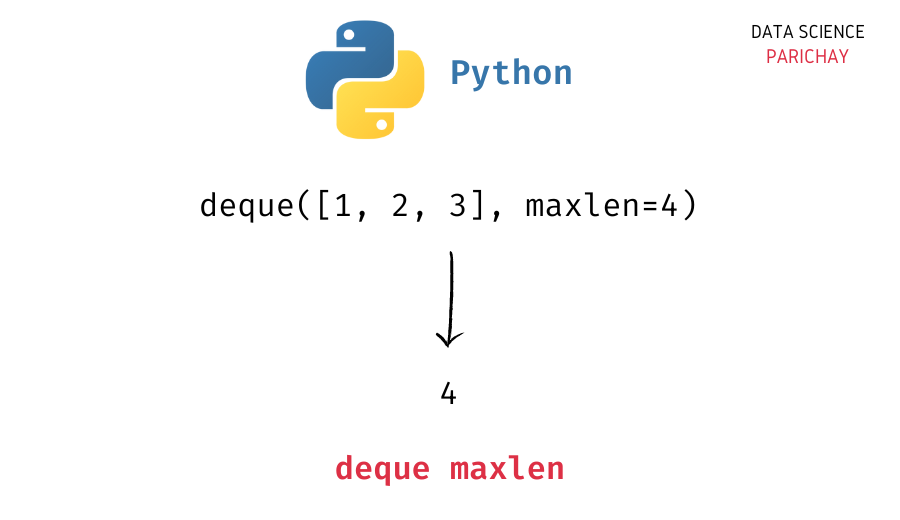# Python – Get the Max Size of a Deque

In this tutorial, we will look at how to get the max size of a deque in Python with the help of some examples.

## `deque` in Python

The `collections` module in Python comes with a `deque` class that you can use to implement a stack or queue data structure in Python. Deques support thread-safe, memory efficient appends and pops from either side of the deque with approximately the same O(1) performance in either direction.

In simple terms, you can think of a `deque` in Python as a list-like data structure but with efficient insertion and removal of items from either end (left and right). This makes them very useful when implementing a stack or queue functionality in Python.

## How to get the max size of a deque in Python?

The `deque` class comes with a number of built-in functions to help perform common operations (like adding and removing elements).

The max size of a deque is defined only for bounded deques (deques with a size limit). To get the max size of a deque in Python, use the deque `maxlen` attribute. The following is the syntax –

```# get the max size of deque
queue.maxlen```

The `maxlen` attribute of a deque is set during its creation. By default, it’s set to `None` (which indicates an unbounded deque) unless specified otherwise (in which case, the deque will be a bounded deque).

## Examples

Let’s now look at some examples of using the above syntax –

### Example 1 – Max size of an unbounded deque

First, let’s create an unbounded deque (deque without a set `maxlen`) with some elements.

📚 Data Science Programs By Skill Level

Introductory

Intermediate ⭐⭐⭐

🔎 Find Data Science Programs 👨‍💻 111,889 already enrolled

Disclaimer: Data Science Parichay is reader supported. When you purchase a course through a link on this site, we may earn a small commission at no additional cost to you. Earned commissions help support this website and its team of writers.

```from collections import deque

# create a deque
queue = deque([1, 2, 3])
# print the deque
print(queue)```

Output:

`deque([1, 2, 3])`

Here, we imported the `deque` class from the `collections` module and created a deque object, `queue` with three elements – 1, 2, and 3.

Now, let’s now get its max size using its `maxlen` attribute.

```# get the max size of deque
print(queue.maxlen)```

Output:

`None`

We get the max size of deque as `None` because the deque under consideration is an unbounded deque. The value of the `maxlen` attribute is `None` for unbounded deques.

### Example 2 – Max size of a bounded deque

Let’s now create a bounded deque (deque object with a specified `maxlen` which defines the maximum number of values that it can store).

```# create a bounded deque
queue = deque([1, 2, 3], maxlen=4)
# print the deque
print(queue)```

Output:

`deque([1, 2, 3], maxlen=4)`

Here, we created a bounded deque with `maxlen` 4 and three elements inside it. Now, let’s get its max size using its `maxlen` attribute.

```# get the max size of deque
print(queue.maxlen)```

Output:

`4`

We get the deque’s max size as 4.

## Length vs the max length of a deque

It’s important to not confuse the `maxlen` (the max size) of deque with its length (which you can get using the Python built-in `len()` function.

The length of a deque is the number of elements in a deque. Both bounded and unbounded deques have a length. The `maxlen` (the max size) of a deque, on the other hand, is defined only for bounded deques and represents the maximum number of values the deque can have.

You might also be interested in –

•